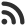Feed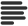Articles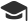Tutorials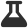Lab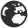CompaniesLeaderboard
 DG Student at BIT Mesra Oct. 12, 2020, 4:47 p.m. ⋅ 867 views

# TCS NQT Technical Questions set 3

61. Recursion is executed in which order :

A. LIFO
B. FIFO
C. Random
D. All of the above

Ans. A

62. Which of the following ADT’s can be used to represent a many-a-many relation ?

A. Tree
B. Stack
C. Graph
D. Queue

Ans. C

63. Merge Sort algorithm is based on which algorithmic technique :

A. D&C
B. Dynamic Programming
C. Greedy
D. Brute Force

Ans. A

64. What will be the time complexity to add an element to the queue?

A. O(1)
B. O(log n)
C. O(n)
D. O(nlogn)

Ans. A

65. A sorting algorithm iteratively traverses through a list to exchange the first element with any element less than it. It then repeats with a new first element. What is this sorting algorithm called ?

A. Insertion Sort
B. Selection Sort
C. Heap Sort
D. Quick Sort

Ans. A

66. The C Processors are specified with _______ symbol.

A. *
B. #
C. \$
D. “

Ans. B

67. A variable declared in a function can be used in main ?

A. True
B. False
C. True if declared static
D. None of the above

Ans. B

68. Which is not a valid C statement :

A. int _t=0;
B. int Main=2;
C. float rate;
D. int \$main;

Ans. D

69. What is the default return type if it is not specified in function definition ?

A. void
B. int
C. double
D. short int

Ans. A

70. A programmer tries to debug a code of 10,000 lines. It is known that there is a logical error in the first 25 lines of the code. Which of the following is an efficient way to debug the code ?

A. Compile and check line by line
B. Use interpreter on the first 25 lines of code
C. Compile and run the entire code
D. None of the above

Ans. B

71. In Stack, when top reaches to -1, the stack is said to be :

A. Overflow
B. Underflow
C. inflow
D. Runtime Error

Ans. B

72. When double is converted to float then the value is ?

A. Truncated
B. Rounded
C. Depends upon compiler
D. None of the above

Ans. C

73. This keyword is used for defining new data type names.

A. docstring
B. typedef
C. both
D. None of them

Ans. B

74. Which precision specifier the number of significant digits ?

A. %g
B. %G
C. %f
D. Both a and b

Ans. D

75. Control can be made out of recursion using :

A. pass
B. break
C. return
D. exit

Ans. C

76. Which of the following does not initialize ptr to null (assume a = 0) ?

A. int *ptr = &a;
B. int *ptr = &a – &a;
C. int *ptr = a – a;
D. All of the above

Ans. A

77. When a function is called by itself again and again, then it is :

A. call by value
B. call by reference
C. recursion
D. System Call

Ans. C

78. Which can never be called by using call by value method :

A. Structure
B. Union
C. Array
D. All of the above

Ans. C

79. What is the maximum number of arguments that can be passed in a single function ?

A. 341
B. 263
C. 253
D. 308

Ans. C

80. In order to display address, we need :

A. %q
B. %a
C. %p
D. None of the mentioned

Ans. C

81. Which header file can be used to define i/o function prototypes and macros?

A. stdio.h
B. conio.h
C. stlib.h
D. alloc.h

Ans. A

82. What is the use of ‘%%’ specifier ?

A. Finding remainder
B. Finding quotient
C. Printing % sign
D. None of the above

Ans. C

83. Which of the following statement is wrong about Java Programming ?

A. Java is compiled and Interpreted
C. Java uses destructors to undo work done by constructors
D. Java is platform independent

Ans. C

84. Which is used to find and fix bugs in the java programs ?

A. JVM
B. JRE
C. JDK
D. JDB

Ans. D

85. Problems associated with dangling pointer can be solved :

A. Assigning it to garbage value
B. Assigning it to NULL value
C. Assigning it to 0
D. None of these

Ans. C

86. Which of the following is a mutable class in Java ?

A. java.lang.String
B. java.lang.Byte
C. java.lang.Short
D. java.lang.StringBuilder

Ans. D

87. Number of threads that can be executed at a time ?

A. 1
B. Multiple
D. 2

Ans. B

88. Which is a wrong keyword as a access modifier ?

A. public
B. default
C. protected
D. private

Ans. B

89. Class X extends Y implements Z shows what kind of a relationship ?

A. X is a Y, X is a Z
B. X has a Z
C. X is a Y, has a Z
D. None of these

Ans. A

90. Which of the following is a reserved keyword in Java?

A. strictfp
B. String
C. main
D. system

Ans. A

Events

Nov. 28, 2018, 5:30 p.m.

Python from zero to hero

place Delhi

Aug. 13, 2018, 5:30 p.m.

Python from zero to hero

place Bangalore ( HackersFriend office BTM Layout)